Processing ......FreeComputerBooks.com Links to Free Computer, Mathematics, Technical Books all over the World

Statistics Done Wrong: The Woefully Complete Guide
Top Free Programming Books 🌠 - 100% Free or Open Source!
• Title Statistics Done Wrong: The Woefully Complete Guide
• Author(s) Alex Reinhart
• Publisher: No Starch Press; 1 edition (2015); eBook (Creative Commons Edition)
• Paperback 176 pages
• eBook HTML
• Language: English
• ISBN-10: 1593276206
• ISBN-13: 978-1593276201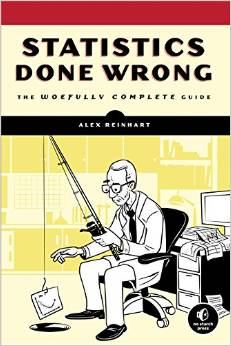Book Description

Scientific progress depends on good research, and good research needs good statistics. But statistical analysis is tricky to get right, even for the best and brightest of us. You'd be surprised how many scientists are doing it wrong.

Statistics Done Wrong is a pithy, essential guide to statistical blunders in modern science that will show you how to keep your research blunder-free. You'll examine embarrassing errors and omissions in recent research, learn about the misconceptions and scientific politics that allow these mistakes to happen, and begin your quest to reform the way you and your peers do statistics.

• Alex Reinhart is an assistant teaching professor of Statistics & Data Science at Carnegie Mellon University, working on statistical models to understand and predict where crimes occur, while also studying how students learn statistics and how we can teach better.
Reviews, Ratings, and Recommendations: Related Book Categories: Read and Download Links:Similar Books:
•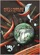Mostly Harmless Statistics (Rachel L. Webb)

This text is for an introductory level probability and statistics course with an intermediate algebra prerequisite. The focus of the text follows the American Statistical Association's Guidelines for Assessment and Instruction in Statistics Education (GAISE).

•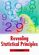Revealing Statistical Principles (Jim K. Lindsey)

Written by a renowned statistician, this book presents the basic ideas behind the statistical methods commonly used in studies of human subjects. Accessible to a wide audience with little background in statistics.

•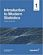Introduction to Modern Statistics (Mine Çetinkaya-Rundel, et al.)

This book puts a heavy emphasis on exploratory data analysis and provides a thorough discussion of simulation-based inference using randomization and bootstrapping, followed by a presentation of the related Central Limit Theorem based approaches.

•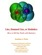Lies, Damned Lies: How to Tell the Truth with Statistics

Help you learn How to Tell the Truth with Statistics and, therefore, how to tell when others are telling the truth ... or are faking their "news". Covers Data Analysis, Binomial and normal models, Sample statistics, confidence intervals, hypothesis tests, etc.

•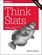Think Stats, 2nd Edition: Exploratory Data Analysis in Python

This concise introduction shows you how to perform statistical analysis computationally, rather than mathematically, with programs written in Python. You'll become familiar with distributions, the rules of probability, visualization, and many other tools and concepts.

•Foundations in Statistical Reasoning (Pete Kaslik)

This book is designed for students taking an introductory statistics class. The emphasis throughout the entire book is on how to make decisions with only partial evidence. It focuses on the thought process.

•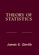Theory of Statistics (James E. Gentle)

This book is directed toward students for whom mathematical statistics is or will become an important part of their lives. It covers classical likelihood, Bayesian, and permutation inference; an introduction to basic asymptotic distribution theory; and modern topics.

•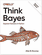O'Reilly® Think Bayes: Bayesian Statistics in Python

If you know how to program, you're ready to tackle Bayesian statistics. With this book, you'll learn how to solve statistical problems with Python code instead of mathematical formulas, using discrete probability distributions rather than continuous mathematics.

•Statistical Inference: Algorithms, Evidence, and Data Science

A masterful guide to how the inferential bases of classical statistics can provide a principled disciplinary frame for the data science of the twenty-first century. Every aspiring data scientist should carefully study this book, use it as a reference.

•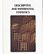Foundations of Descriptive and Inferential Statistics (H. van Elst)

This book aim to provide an accessible though technically solid introduction to the logic of systematical analyses of statistical data to both undergraduate and postgraduate students, in particular in the Social Sciences, Economics, and the Financial Services.

•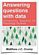Answering Questions with Data : Introductory Statistics

This is a free textbook teaching introductory statistics for undergraduates. Students will learn to select an appropriate data analysis technique, carry out the analysis, and draw appropriate conclusions.

•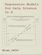Regression Models for Data Science in R (Brian Caffo)

The book gives a rigorous treatment of the elementary concepts of regression models from a practical perspective. The ideal reader for this book will be quantitatively literate and has a basic understanding of statistical concepts and R programming.

•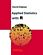Applied Statistics with R (David Dalpiaz)

This book provides an integrated treatment of statistical inference techniques in data science using the R Statistical Software. It provides a much-needed, easy-to-follow introduction to statistics and the R programming language.

•An Introduction to Statistical Learning: with Applications in R

It provides an accessible overview of the field of statistical learning, an essential toolset for making sense of the vast and complex data sets that have emerged in fields ranging from biology to finance to marketing to astrophysics in the past twenty years.

Book Categories
 :All CategoriesTop Free BooksRecent BooksMiscellaneous BooksComputer EngineeringComputer LanguagesComputer ScienceData Science/DatabasesJava and Java EE (J2EE)Linux and UnixMathematicsMicrosoft and .NETMobile ComputingNetworking and CommunicationsSoftware EngineeringSpecial TopicsWeb Programming
Other Categories